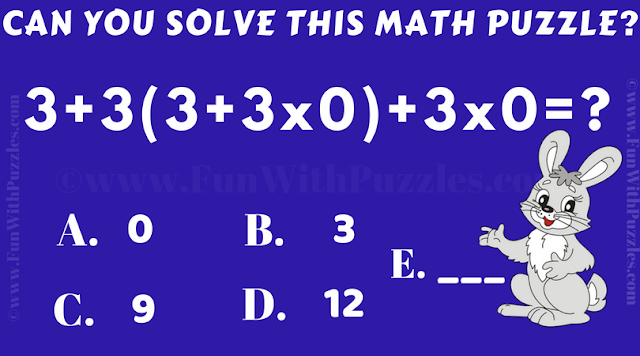This Arithmetic Math Riddle is for teen students going to middle school. This will test your basic Mathematical Knowledge of Arithmetic. In this Cool Math Riddle, there is one Arithmetic Equation that you need to solve. Can you solve this Math Equation correctly on your first attempt?Can you solve this Cool Math Riddle?